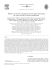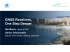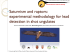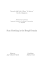# How to calculate a p − value of independence of

## Transcription

How to calculate a p − value of independence of
```Introduction Local correlation Chromosome-scale correlation Genomewide R implementation Conclusions
How to calculate a p − value of independence of
two genome markups?
GenomtriCorr: An attempt of a cookbook
March 24, 2011
Introduction Local correlation Chromosome-scale correlation Genomewide R implementation Conclusions
Outline
Introduction
Introduction itself
Different senses of correlation
Local correlation
Kolmogorov-Smirnov
The sign of correlation
Chromosome-scale correlation
Absolute distance test
Bernoulli test
Na¨ıve Jaccard approach
Genomewide
R implementation
Installation
Usage
Technical
Conclusions
Introduction Local correlation Chromosome-scale correlation Genomewide R implementation Conclusions
A markup
What is it a markup?
What we refer to as a markup is whatever we can represent as a
set of intervals on chromosomes. In other words, it is a spatial
annotation of a genome. It could be any interval annotation on
genome: genes, upstreams, TFBS, clusters, CpG islands, etc...
Introduction Local correlation Chromosome-scale correlation Genomewide R implementation Conclusions
Two markups
Are these two things independent? What does it mean? p − value?
Let’s say one of two markups (query) is independent from the other
markup (reference) if the query is positioned in a manner that is
’blind’ to the scattering of reference. The relation is asymmetric.
Introduction Local correlation Chromosome-scale correlation Genomewide R implementation Conclusions
Different senses of correlation
Chromosome-scale (“global”) negative correlation.
Introduction Local correlation Chromosome-scale correlation Genomewide R implementation Conclusions
Different senses of correlation
Chromosome-scale (“global”) negative correlation.
’Local’ positive correlation.
Introduction Local correlation Chromosome-scale correlation Genomewide R implementation Conclusions
Different senses of correlation
Chromosome-scale (“global”) negative correlation.
’Local’ positive correlation.
Asymmetric relation. Query and reference.
Introduction Local correlation Chromosome-scale correlation Genomewide R implementation Conclusions
Local correlation: contracted intervals
First of all, we contract all the intervals, both query and reference,
into their characteristic points (middles).
Introduction Local correlation Chromosome-scale correlation Genomewide R implementation Conclusions
Local correlation: relative distances and
Kolmogorov-Smirnov
So, relative distance di for a query point i is:
di =
min (|qi − rk | , |rk+1 − qi |)
, k = arg min (qi − rk ).
qi ≥rk
|rk+1 − rk |
If the markups are locally independent, the di ’s are to be uniformly
i.i.d. (u.i.i.d) in [0..0.5]. The corresponding p − value is obtained
by Kolmogorov-Smirnov’s test.
Introduction Local correlation Chromosome-scale correlation Genomewide R implementation Conclusions
Local correlation: The sign of correlation
Blue line: theoretical distribution for
independence (uniform)
Green solid line: they like each other
Green dash line: they dislike each other
Introduction Local correlation Chromosome-scale correlation Genomewide R implementation Conclusions
Local correlation: The sign of correlation
Blue line: theoretical distribution for
independence (uniform)
Green solid line: they like each other
Green dash line: they dislike each other
CorrECDF =
Z
0
0.5
(ECDF (d) − ECDFideal (d)) dd
.
Z 0.5
ECDFideal (d) dd
0
Introduction Local correlation Chromosome-scale correlation Genomewide R implementation Conclusions
Local correlation: The sign of correlation
Blue line: theoretical distribution for
independence (uniform)
Green solid line: they like each other
Green dash line: they dislike each other
CorrECDF =
Z
0
0.5
(ECDF (d) − ECDFideal (d)) dd
.
Z 0.5
ECDFideal (d) dd
0
Positive CorrECDF shows positive local correlation (the distribution
density is shifted towards 0) and vice versa.
Introduction Local correlation Chromosome-scale correlation Genomewide R implementation Conclusions
Local correlation: ECDF area test
Z
0.5
|ECDF (d) − ECDFideal (d)| dd
S=
0
is a measure of discrepancy of real and ideal
ECDF’s.
Introduction Local correlation Chromosome-scale correlation Genomewide R implementation Conclusions
Local correlation: ECDF area test
Z
0.5
|ECDF (d) − ECDFideal (d)| dd
S=
0
is a measure of discrepancy of real and ideal
ECDF’s.
Permutations: drawing N sets of di we get N outcomes for
“null-hypothesis” S and we get p − value for S.
Introduction Local correlation Chromosome-scale correlation Genomewide R implementation Conclusions
Chromosome-scale correlation: Absolute distance test
For each query point i, li = mink (qi − rk ) is found.
L = hli i characterises the “attraction” or “repulsion” of query and
reference points.
Introduction Local correlation Chromosome-scale correlation Genomewide R implementation Conclusions
Chromosome-scale correlation: Absolute distance test
For each query point i, li = mink (qi − rk ) is found.
L = hli i characterises the “attraction” or “repulsion” of query and
reference points.
Permutations: we draw N pseudo-queries as sets of u.i.i.d. points,
calculating “null” for L. The test is two-sided, it gives both
p − value for the real L and the sign of effect if there is one.
Introduction Local correlation Chromosome-scale correlation Genomewide R implementation Conclusions
Chromosome-scale correlation: Bernoulli test
If the coverage of the reference is high, we can use Bernoulli test.
We contract only the query. The probability for a query point to
get into a reference interval is:
p=
coverage of the reference
.
chromosome length
The number of “successes” is approximately Bernoulli with the
parameters #q and p. The test is two-sided; it provides both
p − value and the direction.
Introduction Local correlation Chromosome-scale correlation Genomewide R implementation Conclusions
Chromosome-scale correlation: Na¨ıve Jaccard approach
The coverage is high. Now, both markups are sets of nucleotides.
Jaccard measure (index): J(A, B) =
A∩B
A∪B
Introduction Local correlation Chromosome-scale correlation Genomewide R implementation Conclusions
Chromosome-scale correlation: Na¨ıve Jaccard approach
The coverage is high. Now, both markups are sets of nucleotides.
Jaccard measure (index): J(A, B) =
A∩B
A∪B
Permute the query. Two kinds of permutation a) permute the
starts b)permute the intervals order and permute the gaps order.
Introduction Local correlation Chromosome-scale correlation Genomewide R implementation Conclusions
Genomewide tests
All the test described above are applicable to the genome awhole.
The data for the criteria is summarised over the chromosomes.
The absolute distances are scaled by the expectation of the
distance between adjacent reference points. Then, all the tests are
run for the accumulated data in the same way as it is done for
each chromosome.
Introduction Local correlation Chromosome-scale correlation Genomewide R implementation Conclusions
R implementation
• Based on IRanges
Introduction Local correlation Chromosome-scale correlation Genomewide R implementation Conclusions
R implementation
• Based on IRanges
• Utilities: read test files and visualise IRanges
Introduction Local correlation Chromosome-scale correlation Genomewide R implementation Conclusions
R implementation
• Based on IRanges
• Utilities: read test files and visualise IRanges
• Main procedure
Introduction Local correlation Chromosome-scale correlation Genomewide R implementation Conclusions
R implementation
• Based on IRanges
• Utilities: read test files and visualise IRanges
• Main procedure
• GenomtriCorr package
http://genometricorr.sourceforge.net/
Introduction Local correlation Chromosome-scale correlation Genomewide R implementation Conclusions
Let’s install the package
• In R:
source("http://bioconductor.org/biocLite.R")
biocLite("IRanges")
Introduction Local correlation Chromosome-scale correlation Genomewide R implementation Conclusions
Let’s install the package
• In R:
source("http://bioconductor.org/biocLite.R")
biocLite("IRanges")
• In shell:
R CMD INSTALL GenometriCorr 1.02.tar.gz
Introduction Local correlation Chromosome-scale correlation Genomewide R implementation Conclusions
Let’s install the package
• In R:
source("http://bioconductor.org/biocLite.R")
biocLite("IRanges")
• In shell:
R CMD INSTALL GenometriCorr 1.02.tar.gz
• In R:
library("GenometriCorr")
Introduction Local correlation Chromosome-scale correlation Genomewide R implementation Conclusions
USCSrefseqgenesURL<-’http://genome.ucsc.edu/cgi-bin/hgTables?db=hg19&hgta database=hg19&
hgta group=genes&hgta track=refGene&
hgta table=refGene&hgta regionType=genome&hgta outputType=primaryTable&
hgta fieldSelectTable=hg19.refGene&hgta fs.check.hg19.refGene.chrom=1&hgta fs.check.hg19.refGene.name=1&
hgta fs.check.hg19.refGene.txEnd=1&hgta fs.check.hg19.refGene.txStart=1&hgta doPrintSelectedFields=&’
hgta database=hg19&hgta fieldSelectTable=hg19.cpgIslandExt&hgta fs.check.hg19.cpgIslandExt.chrom=1&
hgta fs.check.hg19.cpgIslandExt.chromEnd=1&hgta fs.check.hg19.cpgIslandExt.chromStart=1&
hgta fs.check.hg19.cpgIslandExt.cpgNum=0&hgta fs.check.hg19.cpgIslandExt.gcNum=0&
hgta fs.check.hg19.cpgIslandExt.length=0&hgta fs.check.hg19.cpgIslandExt.name=0&
hgta fs.check.hg19.cpgIslandExt.obsExp=0&hgta fs.check.hg19.cpgIslandExt.perCpg=0&
hgta fs.check.hg19.cpgIslandExt.perGc=0&hgta group=regulation&hgta outputType=primaryTable&
hgta regionType=genome&hgta table=cpgIslandExt&hgta track=cpgIslandExt&hgta doPrintSelectedFields=&
org=Human&’
Introduction Local correlation Chromosome-scale correlation Genomewide R implementation Conclusions
Utilities: visualise
VisualiseTwoIRanges(cpgis["chr1"]\$ranges, refseq["chr1"]\$ranges, nameA = "CpG Islands", nameB =
"RefSeq Genes", chrom length = human.chrom.length[["chr1"]], title = "CpGIslands and RefGenes on
chr1 of Hg19 animal")
CpGIslands and RefGenes on chr1 of Hg19 animal
CpG Islands
RefSeq Genes
0.0e+00
5.0e+07
1.0e+08
1.5e+08
2.0e+08
2.5e+08
Introduction Local correlation Chromosome-scale correlation Genomewide R implementation Conclusions
Main procedure: GenometricCorrelation
cpgi to genes <- GenometricCorrelation(cpgis, refseq, chromosomes.length = human.chrom.length,
chromosomes.to.proceed = c("chr1"), ecdf.area.permut.number = pn.area,
mean.distance.permut.number = pn.dist, jaccard.measure.permut.number = pn.jacc,
keep.distributions = TRUE, showProgressBar = FALSE)
absolute distances data
1.0
CpGi to Ref Seq Genes, chr 1
0.6
0.8
Query population : 2462
Reference population : 3727
Relative Ks p−value : 5.73992953167846e−09
Relative ecdf deviation area : 0.0205651929973611
Relative ecdf area correlation : 0.0825944507187317
Relative ecdf deviation area p−value : <0.01
Scaled Absolute min. distance p−value : <0.01
Jaccard Measure p−value : <0.01
Jaccard Measure lower tail : FALSE
0.0
0.2
0.4
Fn(x)
0.6
0.4
0.2
0.0
twotimes (x)
0.8
1.0
relative distances data
0.0
0.1
0.2
0.3
x
0.4
0.5
0
200000 400000 600000 800000
x
1200000
Introduction Local correlation Chromosome-scale correlation Genomewide R implementation Conclusions
Some technical issues:R
• In R:
package.skeleton()
Introduction Local correlation Chromosome-scale correlation Genomewide R implementation Conclusions
Some technical issues:R
• In R:
package.skeleton()
• In shell:
R CMD check GenometriCorr
R CMD build GenometriCorr
Introduction Local correlation Chromosome-scale correlation Genomewide R implementation Conclusions
Some technical issues:Documentation
• In R:
Sweave(’GenometricCorrelationPackage.Rnw’)
In shell:
R CMD Sweave GenometricCorrelationPackage
Introduction Local correlation Chromosome-scale correlation Genomewide R implementation Conclusions
Some technical issues:Documentation
• In R:
Sweave(’GenometricCorrelationPackage.Rnw’)
In shell:
R CMD Sweave GenometricCorrelationPackage
• In shell:
echo "library(weaver);
Sweave(’GenometricCorrelationPackage.Rnw’,
driver=weaver())" | R --no-save --no-restore
Introduction Local correlation Chromosome-scale correlation Genomewide R implementation Conclusions
Alexander Favorov
Loris Mularoni
Leslie Cope
Yulia Medvedeva
Vsevolod Makeev
Sarah Wheelan
Introduction Local correlation Chromosome-scale correlation Genomewide R implementation Conclusions
Conclusions
```

### gigasecond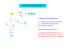### History of Mazda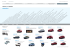### Analisis Pengaruh Atmosfer Waroeng Joglo “Bu Rini” terhadap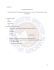### KUISIONER Kepada, Siswa/i Lembaga Bimbingan Belajar SSC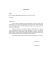### from enea.it